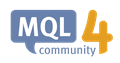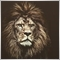# Is My Understanding of the 'NormalizeDouble' Function Correct?448

This code is straight from page (https://book.mql4.com/operators/switch) of the manual.

```int start()                                     // Special function 'start'
{
double Level=1.3200;                           // Preset price level
int Delta=NormalizeDouble((Bid-Level)Point,0); // Excess
if (Delta<=0)                                  // Price doesn't excess the level
{
Alert("The price is below the level");      // Message
return;                                     // Exit start()
}
//------------------------------------------------------------------------------------
```

I am a little confused over the format of the line:

`int Delta=NormalizeDouble((Bid-Level)Point,0); // Excess `

I understand that 'NormalizeDouble' is a rounding tool that is used to round a number to a specified amount of decimal places.

I understand that 'Point' or 'point value' or 'point size' is effectively a tenth of a pip.

I understand that the '0' represents the number of decimal places that 'NormalizeDouble' should round to.

But am I correct in saying that the mentioned line effectively assigns 'Delta' with the point value of (Bid-Level) to 0 decimal places? Or am I totally along the wrong lines?

----------------

e.g. if:

Bid=1.3201
and
Level=1.3200

then:

int Delta=10

----------------Operator 'switch' - Operators - MQL4 Tutorial
• book.mql4.com
Some programs imply branching of their algorithm into several variations. In such cases, it is very convenient to use the operator 'switch', especially if there are tens or hundreds of variations, whereas the 'if' code becomes bloated if you use many nested operators. Format of the Operator 'switch' The operator 'switch' consists of the header...9829

koranged:

This code is straight from page (https://book.mql4.com/operators/switch) of the manual.

I am a little confused over the format of the line:

It normalizes the double up to a defined no. of digits..
also it should be

`int Delta=NormalizeDouble((Bid-Level)/Point,0); // Excess `

and to make that warning go away you should convert it to int

`int Delta= (int)NormalizeDouble((Bid-Level)/Point,0); // Excess `448

Lakshan Perera:

It normalizes the double up to a defined no. of digits..
also it should be

and to make that warning go away you should convert it to int

Hi Lakshan,

This is an example taken directly from the manual. It's not a code that I've programmed myself, I am just trying to understand the functionality of this particular example.

This is exactly how the line is from the manual:

`int Delta=NormalizeDouble((Bid-Level)Point,0); // Excess `

So in the above example, would I be correct in saying that;  'Delta' is assigned with the point value of (Bid-Level) to 0 decimal places?

Or are you trying to say that the line does not make sense unless there is a division (/) in between (Bid-Level) and Point?

Thanks again.9829

koranged:

Hi Lakshan,

This is an example taken directly from the manual. It's not a code that I've programmed myself, I am just trying to understand the functionality of this particular example.

This is exactly how the line is from the manual:

So in the above example, would I be correct in saying that;  'Delta' is assigned with the point value of (Bid-Level) to 0 decimal places?

Or are you trying to say that the line does not make sense unless there is a division (/) in between (Bid-Level) and Point?

Thanks again.

1) NormalizeDouble() with 0 digits, is a sort of rounding off to value without any decimal place(aka 0 digits after the decimal point) ==> integer (as mentioned above)
2) Also, if you try to compile the above code without the division operator(/) or any other operator for that matter, you will encounter an error.. 'Point' - some operator expected448

Lakshan Perera:

1) NormalizeDouble() with 0 digits, is a sort of rounding off to value without any decimal place ==> integer (as mentioned above)
2) Also, if you try to compile the above code without the division operator(/) or any other operator(even though it is incorrect) you will encounter an error..

I'm not trying to compile this code... this is just an example from the manual. I am not going to be using this EA myself it is just simply an example from the manual of how a 'Switch' operator works, which happens to use that line within it.

I understand that NormalizeDouble() with 0 digits is rounding it off without a decimal place.

Are you saying that the following code:

`int Delta=NormalizeDouble((Bid-Level)Point,0); // Excess `

Does not make sense? As I've mentioned this is from the MQL5 Manual Tutorial so I doubt they would have left an incorrect example in there...

------------------------------------------------------------------------------------------------------------------------------------------------------------------------------------------------------------------------------

But let's say that I put that division sign in there to make the code look like this:

`int Delta=NormalizeDouble((Bid-Level)/Point,0); // Excess `

Will that then divide the difference between the bid and level with the current point value?

And if so:

How is the point value displayed? If EUR/USD is trading exactly at 1.17002 then does this display 'point' as:

1.17002

or

2

Thanks again.9829

koranged:

I'm not trying to compile this code... this is just an example from the manual. I am not going to be using this EA myself it is just simply an example from the manual of how a 'Switch' operator works, which happens to use that line within it.

I understand that NormalizeDouble() with 0 digits is rounding it off without a decimal place.

Are you saying that the following code:

Does not make sense? As I've mentioned this is from the MQL5 Manual Tutorial so I doubt they would have left an incorrect example in there...

Yes it is wrong without any operator..

koranged:

But let's say that I put that division sign in there to make the code look like this:

Will that then divide the difference between the bid and level with the current point value?

And if so:

How is the point value displayed? If EUR/USD is trading exactly at 1.17002 then does this display 'point' as:

1.17002

or

2

Thanks again.

in your example value of Point is 0.00001448

Lakshan Perera:

Yes it is wrong without any operator..

in your example value of Point is 0.00001

I think I understand what you are saying now.

So if I add the division sign to the example and we presume that EUR/USD is Bid at 1.3201, and 'Level' maintains the value of '1.3200':

`int Delta= (int)NormalizeDouble((Bid-Level)/Point,0);`

...then 'Delta' effectively assumes the value of 10 due to the calculation:

`(1.3201-1.3200)/0.00001 = 10`

Is this correct?9829

koranged:

I think I understand what you are saying now.

So if I add the division sign to the example and we presume that EUR/USD is Bid at 1.3201:

...then 'Delta' effectively assumes the value of 10 due to the calculation:

Is this correct?

Yes exactly.. :-)448

Lakshan Perera:

Yes exactly.. :-)

Great!

Also I just downloaded the .mq4 file of the example they gave on the tutorial and in the actual code the division sign is included, so I guess if I had just checked there then could have avoided all the confusion!

Thanks a lot for your help Lakshan.20208

Do NOT use NormalizeDouble, EVER. For ANY Reason. It's a kludge, don't use it. It's use is usually wrong, as it is in this case.

Your
`int Delta= (int)NormalizeDouble((Bid-Level)/Point,0);`
is exactly (minus sub-point rounding,) the same as
`int Delta= int((Bid-Level)/Point);`448

whroeder1:
Youris exactly (minus sub-point rounding,) the same as